# RD Sharma Solutions For Class 7 Maths Chapter 23 Data Handling - II (Central Values) Exercise 23.4

Students can view and download the RD Sharma Solutions for Class 7 Maths Exercise 23.4 of Chapter 23 Data Handling – II from the links provided below. The syllabus given by the CBSE for all the classes is completely based on the RD Sharma textbook. Hence, preparation of exams using the RD Sharma Solutions for Class 7 will definitely provide students with an advantage of scoring well. This exercise explains the mode of a group of observations.

## Download the PDF of RD Sharma Solutions For Class 7 Maths Chapter 23 – Data Handling – II (Central Values) Exercise 23.4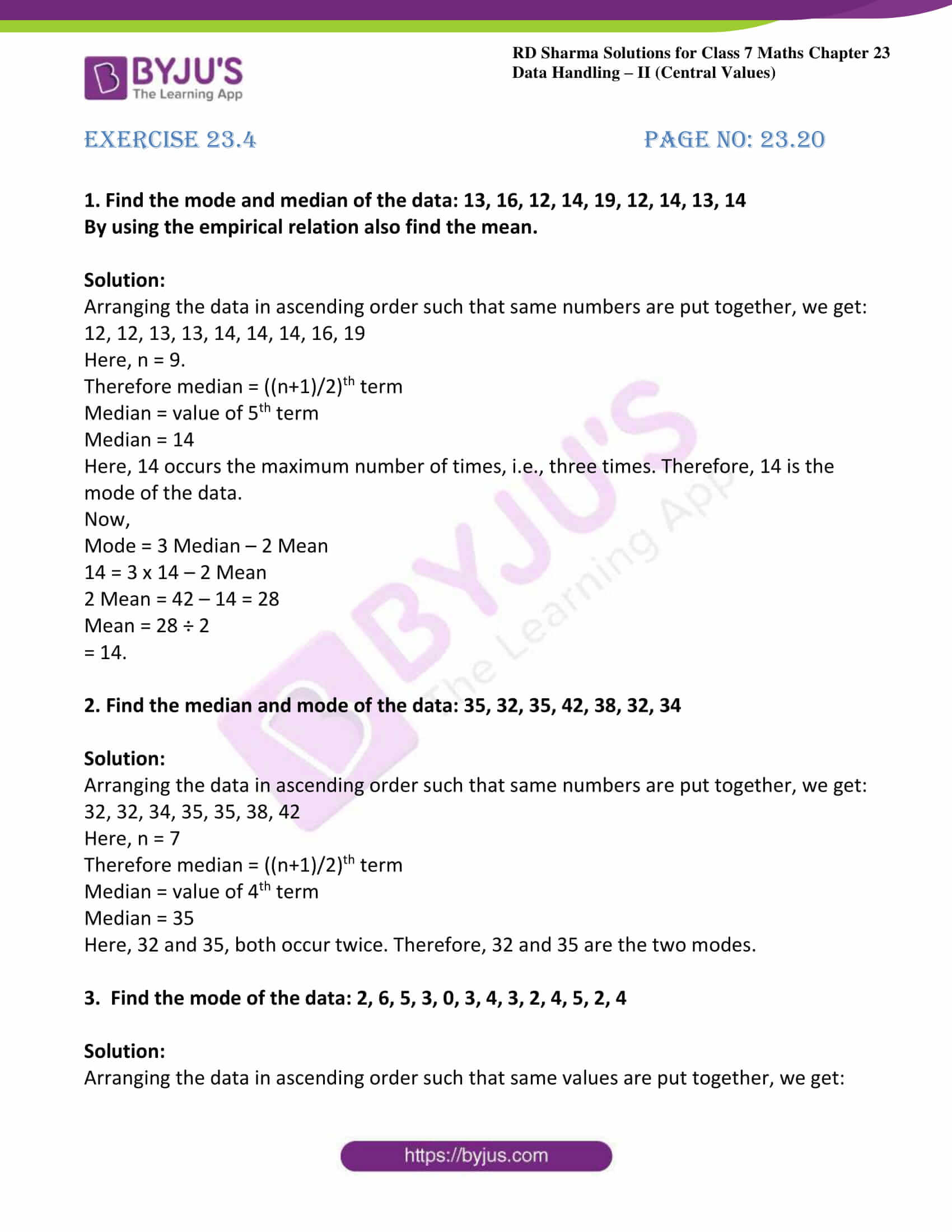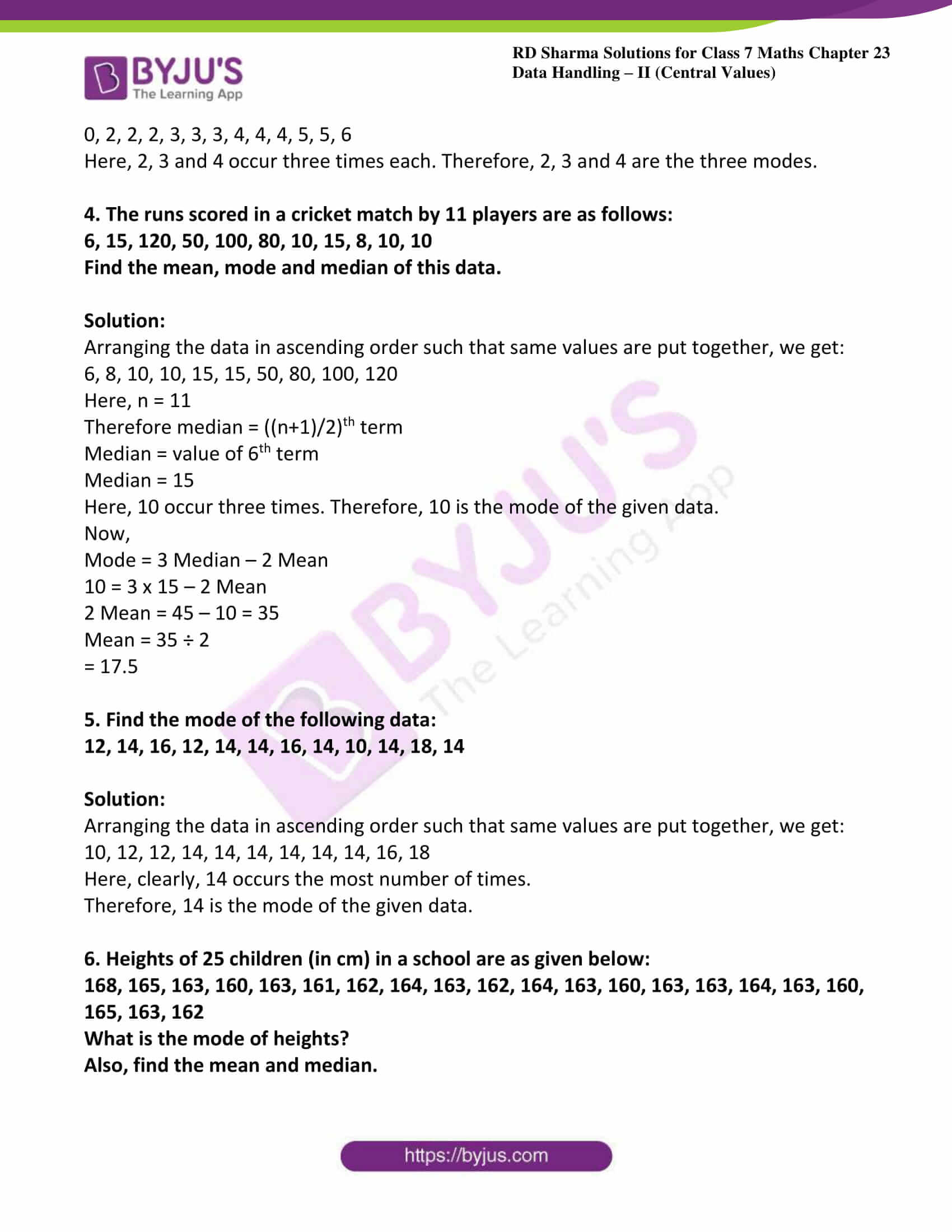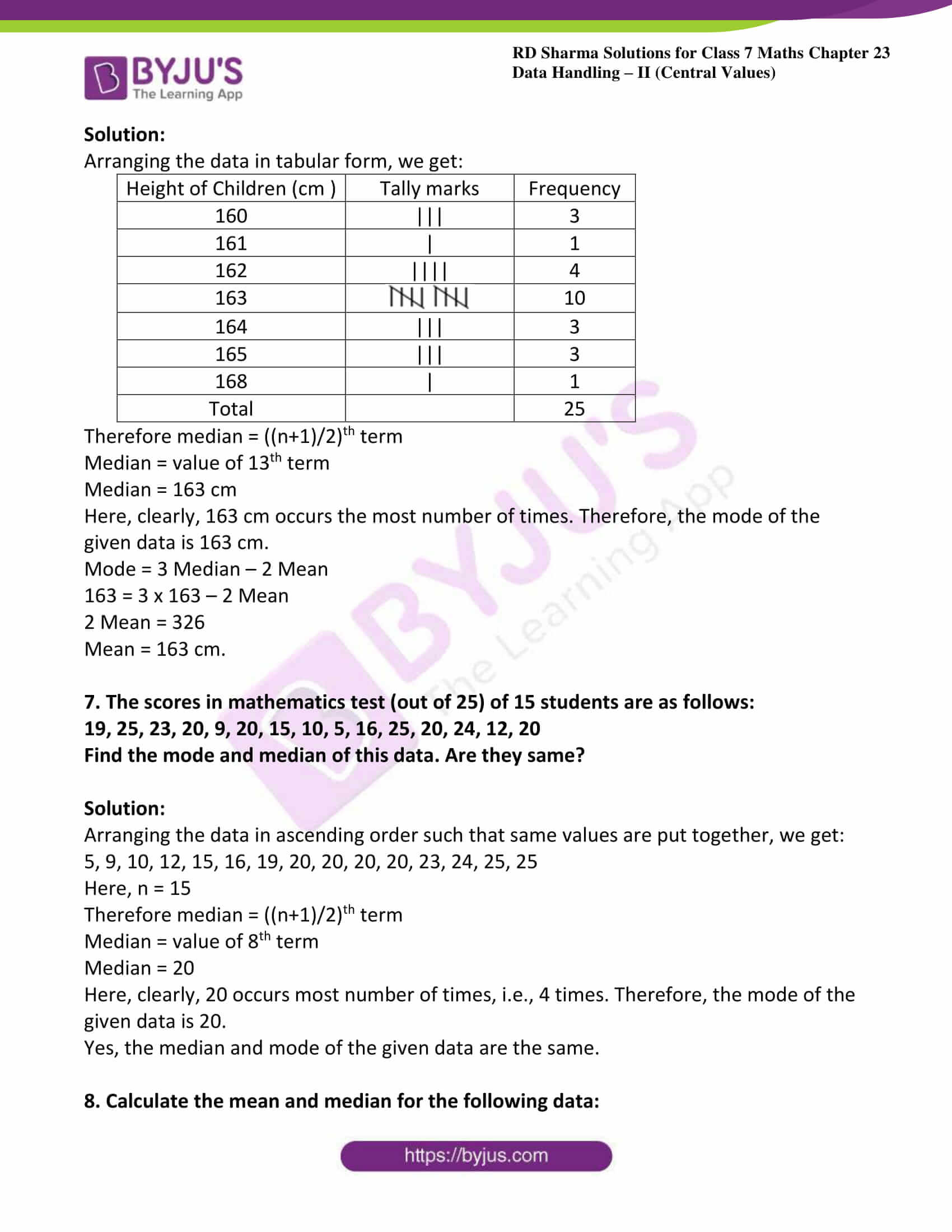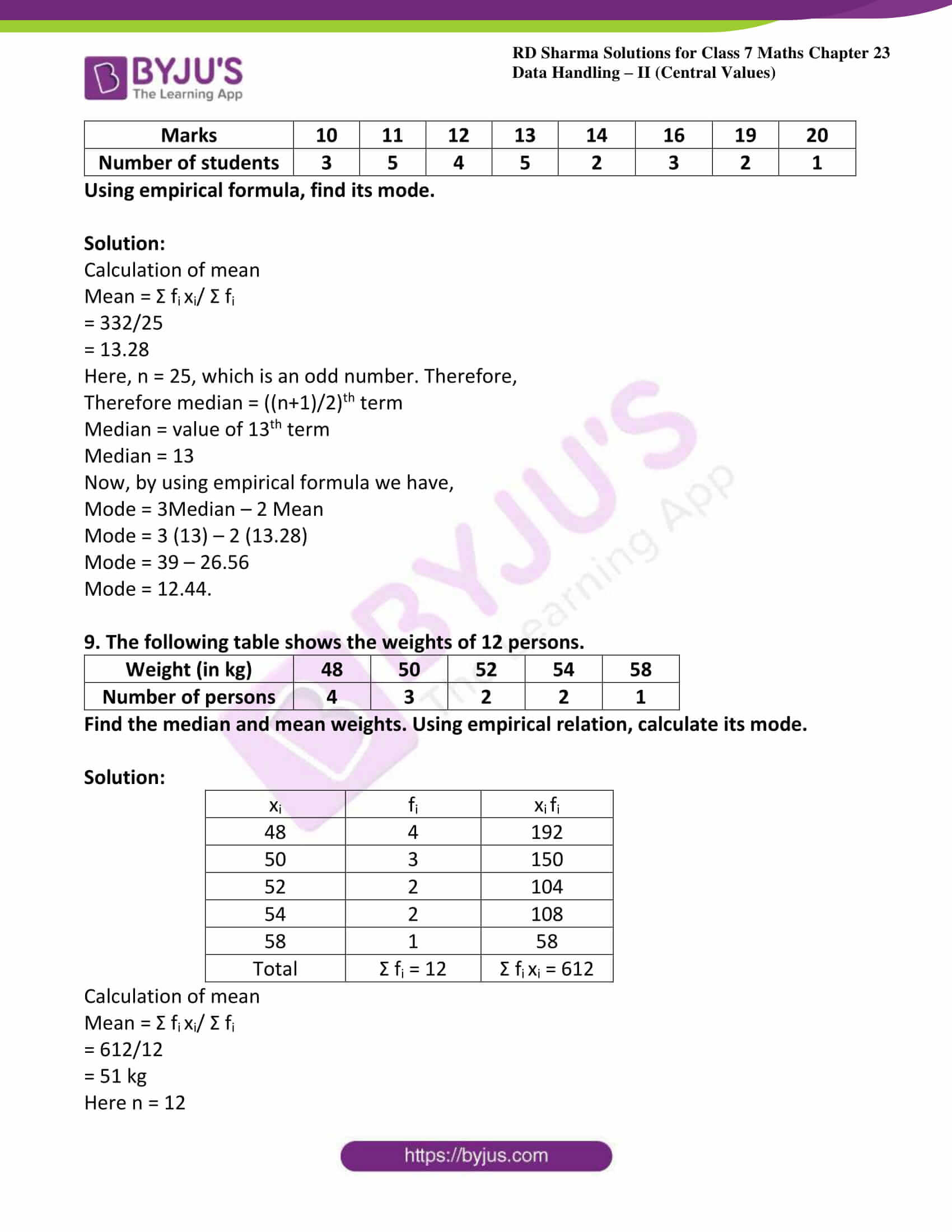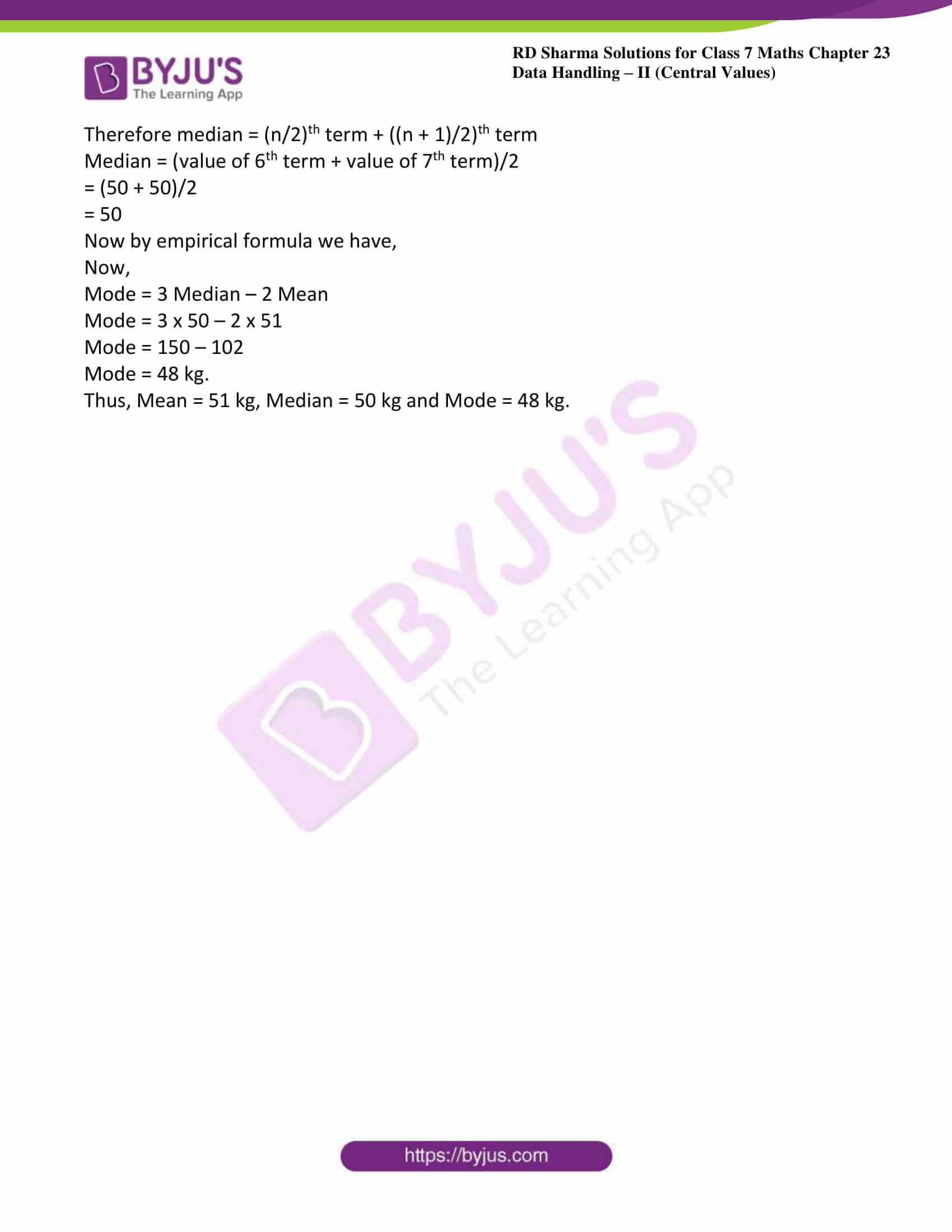### Access answers to Maths RD Sharma Solutions For Class 7 Chapter 23 – Data Handling – II (Central values) Exercise 23.4

1. Find the mode and median of the data: 13, 16, 12, 14, 19, 12, 14, 13, 14

By using the empirical relation also find the mean.

Solution:

Arranging the data in ascending order such that same numbers are put together, we get:

12, 12, 13, 13, 14, 14, 14, 16, 19

Here, n = 9.

Therefore median = ((n+1)/2)th term

Median = value of 5th term

Median = 14

Here, 14 occurs the maximum number of times, i.e., three times. Therefore, 14 is the mode of the data.

Now,

Mode = 3 Median – 2 Mean

14 = 3 x 14 – 2 Mean

2 Mean = 42 – 14 = 28

Mean = 28 ÷ 2

= 14.

2. Find the median and mode of the data: 35, 32, 35, 42, 38, 32, 34

Solution:

Arranging the data in ascending order such that same numbers are put together, we get:

32, 32, 34, 35, 35, 38, 42

Here, n = 7

Therefore median = ((n+1)/2)th term

Median = value of 4th term

Median = 35

Here, 32 and 35, both occur twice. Therefore, 32 and 35 are the two modes.

3.  Find the mode of the data: 2, 6, 5, 3, 0, 3, 4, 3, 2, 4, 5, 2, 4

Solution:

Arranging the data in ascending order such that same values are put together, we get:

0, 2, 2, 2, 3, 3, 3, 4, 4, 4, 5, 5, 6

Here, 2, 3 and 4 occur three times each. Therefore, 2, 3 and 4 are the three modes.

4. The runs scored in a cricket match by 11 players are as follows:

6, 15, 120, 50, 100, 80, 10, 15, 8, 10, 10

Find the mean, mode and median of this data.

Solution:

Arranging the data in ascending order such that same values are put together, we get:

6, 8, 10, 10, 15, 15, 50, 80, 100, 120

Here, n = 11

Therefore median = ((n+1)/2)th term

Median = value of 6th term

Median = 15

Here, 10 occur three times. Therefore, 10 is the mode of the given data.

Now,

Mode = 3 Median – 2 Mean

10 = 3 x 15 – 2 Mean

2 Mean = 45 – 10 = 35

Mean = 35 ÷ 2

= 17.5

5. Find the mode of the following data:

12, 14, 16, 12, 14, 14, 16, 14, 10, 14, 18, 14

Solution:

Arranging the data in ascending order such that same values are put together, we get:

10, 12, 12, 14, 14, 14, 14, 14, 14, 16, 18

Here, clearly, 14 occurs the most number of times.

Therefore, 14 is the mode of the given data.

6. Heights of 25 children (in cm) in a school are as given below:

168, 165, 163, 160, 163, 161, 162, 164, 163, 162, 164, 163, 160, 163, 163, 164, 163, 160, 165, 163, 162

What is the mode of heights?

Also, find the mean and median.

Solution:

Arranging the data in tabular form, we get:

 Height of Children (cm ) Tally marks Frequency 160 ||| 3 161 | 1 162 |||| 4 163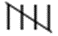10 164 ||| 3 165 ||| 3 168 | 1 Total 25

Therefore median = ((n+1)/2)th term

Median = value of 13th term

Median = 163 cm

Here, clearly, 163 cm occurs the most number of times. Therefore, the mode of the given data is 163 cm.

Mode = 3 Median – 2 Mean

163 = 3 x 163 – 2 Mean

2 Mean = 326

Mean = 163 cm.

7. The scores in mathematics test (out of 25) of 15 students are as follows:

19, 25, 23, 20, 9, 20, 15, 10, 5, 16, 25, 20, 24, 12, 20

Find the mode and median of this data. Are they same?

Solution:

Arranging the data in ascending order such that same values are put together, we get:

5, 9, 10, 12, 15, 16, 19, 20, 20, 20, 20, 23, 24, 25, 25

Here, n = 15

Therefore median = ((n+1)/2)th term

Median = value of 8th term

Median = 20

Here, clearly, 20 occurs most number of times, i.e., 4 times. Therefore, the mode of the given data is 20.

Yes, the median and mode of the given data are the same.

8. Calculate the mean and median for the following data:

 Marks 10 11 12 13 14 16 19 20 Number of students 3 5 4 5 2 3 2 1

Using empirical formula, find its mode.

Solution:

Calculation of mean

Mean = Σ fi xi/ Σ fi

= 332/25

= 13.28

Here, n = 25, which is an odd number. Therefore,

Therefore median = ((n+1)/2)th term

Median = value of 13th term

Median = 13

Now, by using empirical formula we have,

Mode = 3Median – 2 Mean

Mode = 3 (13) – 2 (13.28)

Mode = 39 – 26.56

Mode = 12.44.

9. The following table shows the weights of 12 persons.

 Weight (in kg) 48 50 52 54 58 Number of persons 4 3 2 2 1

Find the median and mean weights. Using empirical relation, calculate its mode.

Solution:

 xi fi xi fi 48 4 192 50 3 150 52 2 104 54 2 108 58 1 58 Total Σ fi = 12 Σ fi xi = 612

Calculation of mean

Mean = Σ fi xi/ Σ fi

= 612/12

= 51 kg

Here n = 12

Therefore median = (n/2)th term + ((n + 1)/2)th term

Median = (value of 6th term + value of 7th term)/2

= (50 + 50)/2

= 50

Now by empirical formula we have,

Now,

Mode = 3 Median – 2 Mean

Mode = 3 x 50 – 2 x 51

Mode = 150 – 102

Mode = 48 kg.

Thus, Mean = 51 kg, Median = 50 kg and Mode = 48 kg.